# Abstract

Empirical dynamic modeling (EDM) is an emerging non-parametric framework for modeling nonlinear dynamic systems. EDM is based on the mathematical theory of recontructing attractor manifolds from time series data (Takens 1981). The rEDM package collects several EDM methods, including simplex projection (Sugihara and May 1990), S-map (Sugihara 1994), multivariate embeddings (Dixon et al. 1999), convergent cross mapping (Sugihara et al. 2012), and multiview embedding (Ye and Sugihara 2016). Here, we introduce the basic underlying theory, and describe the functionality of the rEDM, using examples from both model simulations and real data.

# Introduction

Many scientific fields use modesl as approximations of reality and for various purposes (e.g. testing hypotheses regarding mechanisms or processes, explaining past observations, predicting future outcomes). In most cases these models are based on hypothesized parametric equations; however explicit equations can be impractical when the exact mechanisms are unknown or too complex to be characterized with existing datasets. Empirical models, that infer patterns and associations from the data (instead of using hypothesized equations), represent an alternative and highly flexible approach. Here, we review the theoretical background for the emerging framework of empirical dynamic modeling (EDM) and the functionality of the rEDM package, which are intended for the study nonlinear dynamic systems that can prove problematic for traditional modeling approaches.

The basic goal underlying EDM is to reconstruct the behavior of dynamic systems using time series data. This approach is based on mathematical theory developed initially by (Takens 1981), and expanded on by others (Casdagli et al. 1991, Sauer et al. 1991, Deyle and Sugihara 2011). Because these methods operate with minimal assumptions, they are particularly suitable for studying systems that exhibit non-equilibrium dynamics and nonlinear state-dependent behavior (i.e. where interactions change over time and as a function of the system state).

## Installation Instructions

The rEDM package can be obtained in two main ways. The standard version of the package can be obtained through CRAN (the Comprehensive R Archive Network): https://cran.r-project.org/package=rEDM:

install.packages("rEDM")

However, the most recent version is available on GitHub: https://github.com/ha0ye/rEDM, and can be installed using the install_github function in the devtools package.

devtools::install_github("ha0ye/rEDM")

# Empirical Dynamic Modeling

## Time Series as Observations of a Dynamic System

The essential concept is that time series can be viewed as projections of the behavior of a dynamic system. Here, only a few modest assumptions are required. First, that the system state can be described as a point in a high-dimensional space. The axes of this space can be thought of as fundamental state variables; in an ecosystem, these variables might correspond to population abundances, resources, or environmental conditions. Second, that the system state changes through time following a set of deterministic rules. In other words, the behavior of the system is not completely stochastic.

Consequently, it is possible to project the system state onto one of the coordinate axes to obtain the value of the corresponding state variable. Thus, sequential projections over time will form a time series for that variable. For example, in Figure , the states of the canonical Lorenz Attractor (Lorenz 1963) are projected to the $$x$$-axis, creating a time series for variable $$x$$.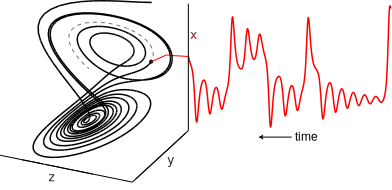Time Series Projection from Lorenz Attractor

Although different time series observed from the system can represent different state variables, in general, each time series is a function of the system state and may potentially convolve several different state variables.

## Attractor Reconstruction / Takens’ Theorem

The goal of EDM is to reconstruct the system dynamics from time series data. As seen above, a time series can be thought of as sequential projections of the motion on an attractor; in other words, information about the behavior is encoded in the temporal ordering of the time series. Takens’ Theorem (Takens 1981) states that mathematically valid and equivalent reconstructions of the attractor can be created using lags of just a single time series, by substituting those lags for unknown or unobserved variables. In other words, instead of representing the system state using a complete set of state variables, we can instead use a lagged-coordinate embedding: $\vec{x}_t = \langle x_t, x_{t-\tau}, \dots, x_{t-(E-1)\tau} \rangle$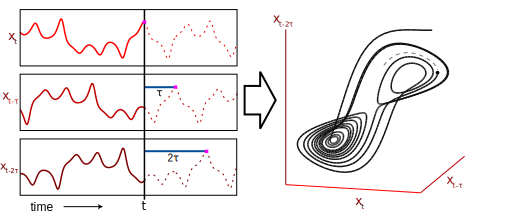Attractor Reconstruction

If sufficient lags are used, the reconstruction preserves essential mathematical properties of the original system: reconstructed states will map one-to-one to actual system states, and nearby points in the reconstruction will correspond to similar system states. Figure shows a reconstruction of the Lorenz attractor (from Figure ) where the reconstructed system state is comprised of 3 lags of variable $$x$$. Here, the visual similarity between the reconstruction and the original Lorenz Attractor is quite clear.

As a consequence of the fact that these mathematical properties of the original system can be recovered from a single time series, there are multiple applications. For example, empirical models can be used for forecasting (Sugihara and May 1990), to understand nonlinear behavior (Sugihara 1994), or to uncover mechanism (Dixon et al. 1999). Moreover, recent work describes how EDM can be used to identify causal interactions, by testing whether two time series are observed from the same system (Sugihara et al. 2012). In the next section, we demonstrate how the rEDM software package can be used to accomplish these various tasks.

# Demonstration of EDM

## Nearest Neighbor Forecasting using Simplex Projection

As mentioned previously, the reconstruction will map one-to-one to the original attractor manifold if enough lags are used (i.e. if the reconstruction has a sufficiently large embedding dimension). If the embedding dimension is too small, then reconstructed states will overlap and appear to be the same even though they actually correspond to different states. These “singularities” will result in poor forecast performance, because the system behavior cannot be uniquely determined in the reconstruction. Thus, we can use prediction skill as an indicator for identifying the optimal embedding dimension. In the following example, we demonstrate how this can be accomplished using a nearest neighbor forecastaing method, Simplex Projection (Sugihara and May 1990), implemented in rEDM as the function simplex.

### Example

In this example, we use simulated time series from the classical tent map that exhibits chaotic behavior. The tent map is a discrete time dynamic system, where a sequence, $$x_t$$, on the interval $$[0, 1]$$ is iterated according to:

$\begin{equation*} x_{t+1} = \begin{cases} 2x_t & x_t < \frac{1}{2}\\ 2(1-x_t) & x_t \ge \frac{1}{2} \end{cases} \end{equation*}$

In rEDM, a sample time series of the first-differenced values can be found in dataset tentmap_del.

library(rEDM)
data(tentmap_del)
str(tentmap_del)
##  num [1:999] -0.0992 -0.6013 0.7998 -0.7944 0.798 ...

We can see that the data consists of just a single vector, containing the raw first-differences values of $$x_t$$. Because the simplex function can accept a single vector as the input time series, no further processing of the data is required.

ts <- tentmap_del
lib <- c(1, 100)
pred <- c(201, 500)

We begin by initializing the lib and pred variables. These determine which portions of the data will be used to create the reconstruction, and which portions of the data the reconstruction will be used to make forecasts on. In other words, defining the “training” and “test” subsets of the data. Here, the first 100 points (rows 1 to 100) in the time series constitute the “library”, and 300 points (rows 201 to 500) are the “prediction set” for which the model will produce forecasts.

The remaining parameters will be left at their default values (see section for details). For the simplex function, this means that the embedding dimension, $$E$$, will range from $$1$$ to $$10$$.

Note that if the code detects any overlap in the lib and pred, it will enable leave-one-out cross-validation and return a warning message.

simplex_output <- simplex(ts, lib, pred)
str(simplex_output)
## 'data.frame':    10 obs. of  16 variables:
##  $E : int 1 2 3 4 5 6 7 8 9 10 ##$ tau                : num  1 1 1 1 1 1 1 1 1 1
##  $tp : num 1 1 1 1 1 1 1 1 1 1 ##$ nn                 : num  2 3 4 5 6 7 8 9 10 11
##  $num_pred : num 299 298 297 296 295 294 293 292 291 290 ##$ rho                : num  0.847 0.962 0.943 0.91 0.874 ...
##  $mae : num 0.207 0.104 0.138 0.19 0.235 ... ##$ rmse               : num  0.392 0.189 0.235 0.291 0.334 ...
##  $perc : num 0.853 0.906 0.899 0.885 0.824 ... ##$ p_val              : num  2.67e-102 9.18e-251 2.13e-200 1.54e-151 2.59e-118 ...
##  $const_pred_num_pred: num 299 298 297 296 295 294 293 292 291 290 ##$ const_pred_rho     : num  -0.668 -0.671 -0.671 -0.673 -0.671 ...
##  $const_pred_mae : num 1.02 1.02 1.02 1.02 1.01 ... ##$ const_pred_rmse    : num  1.25 1.25 1.26 1.26 1.25 ...
##  $const_pred_perc : num 0.341 0.339 0.337 0.338 0.339 ... ##$ const_p_val        : num  1 1 1 1 1 1 1 1 1 1

The returned object from simplex is a simple data.frame with columns for each of the model parameters and forecast statistics, and rows for each separate model (i.e. different parameter combinations). For simplex, the model parameters are E, embedding dimension; tau, time lag between successive dimensions; tp, time to prediction; and nn, number of nearest neighbors (see section for a detailed description). The forecast statistics are num_pred, the number of predictions made; rho, Pearson’s correlation coefficient between predictions and observations; mae, mean absolute error of predictions; rmse, root mean squared error of predictions; perc, the percent of predictions that are the same sign as observations; and p_val, the p-value for rho being significantly greater than 0, using Fisher’s transformation (Fisher 1915). The last 6 columns give those same forecast statistics, but for a naive constant predictor (where the forecast is simply the current value) over the same set of predictions.

In this case, there are 10 separate models (one for each value of E), so we can simply plot E against rho (the correlation between observed and predicted values) to determine the optimal embedding dimension (i.e. the number of dimensions for which the reconstructed attractor is best unfolded, producing the highest forecast skill).

par(mar = c(4, 4, 1, 1), mgp = c(2.5, 1, 0))  # set up margins for plotting
plot(simplex_output$E, simplex_output$rho, type = "l", xlab = "Embedding Dimension (E)",
ylab = "Forecast Skill (rho)")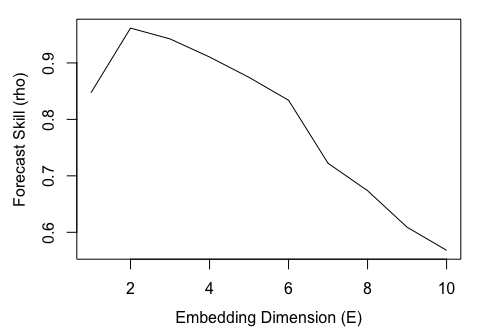Here, we observe that forecast skill peaks at $$E = 2$$, indicating that the dynamics of our data are unfolded best in 2 dimensions. Note that this optimal value for E may not be the same as the actual dimensionality of the corresponding dynamic systems, since predictability will be limited by observational error, process noise, and time series length.

## Prediction Decay

An important property of many natural systems is that nearby trajectories eventually diverge over time (i.e. “deterministic chaos” or the so-called “butterfly effect”). In essence, this means that short-term prediction is possible, because the near future is well-constrained, but in the long-term, states of the system are essentially random and not predictable. We can demonstrate this effect by examining how prediction skill changes as the tp parameter is increased; recall that this parameter describes the forecast horizon for how far ahead into the future forecasts are made.

### Example

Using the same tent map time series, we supply a range for the tp parameter in the simplex function, but fix the embedding dimension to the value determined above ($$E = 2$$):

simplex_output <- simplex(ts, lib, pred, E = 2, tp = 1:10)

As above, the returned object is a data.frame, and the prediction decay can be studied by plotting forecast skill (rho) against the time to prediction (tp).

par(mar = c(4, 4, 1, 1))
plot(simplex_output$tp, simplex_output$rho, type = "l", xlab = "Time to Prediction (tp)",
ylab = "Forecast Skill (rho)")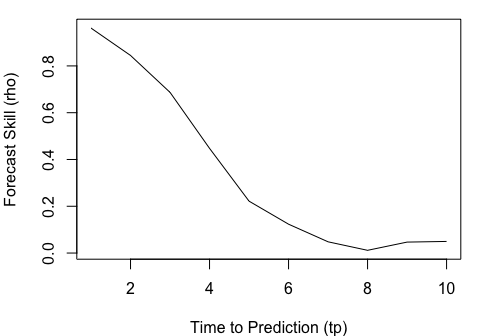As expected (because the parameters chosen for the tent map fall in the region for chaotic behavior), the clear decline in forecast skill (rho $$\rightarrow 0$$) indicates that the system is chaotic.

## Identifying Nonlinearity

One concern is that many time series may show predictability even if they are purely stochastic, because they behave similarly to autocorrelated red noise. Fortunately, we can distinguish between red noise and nonlinear deterministic behavior by using S-maps as described in (Sugihara 1994).

In contrast to the nearest-neighbor interpolation of simplex projection, the S-map forecasting method (Sugihara 1994) fits local linear maps to describe the dynamics. In addition to the standard set of parameters for a lagged-coordinate reconstruction (as in simplex), S-maps also contain a nonlinear tuning parameter, $$\theta$$, that determines the degree to which points are weighted when fitting the local linear map. For example, when $$\theta = 0$$, all points are equally weighted, such that the local linear map is identical for different points in the reconstructed state-space. As such, the S-map will be identical to a global linear map (i.e. an autoregressive model). When values of $$\theta$$ are greater than $$0$$, nearby points in the state space receive larger weights, and the local linear map can vary in state-space to accommodate nonlinear behavior.

Consequently, if the time series are sampled from autoregressive red noise, then the linear model ($$\theta = 0$$) should produce better forecasts, because the global linear map (which will, in effect, be fitted to more data points) will reduce the effects of observation error compared to local linear maps. In contrast, if forecast skill increases for $$\theta > 0$$, then the results are suggestive of nonlinear dynamics, because better forecasts are achieved when the local linear map can change depending on the location in state-space: it is a better description of state-dependent behavior.

### Example

The S-map method is implemented as the function s_map in the rEDM package. Much like in the previous use of simplex, we can leave many of the parameters at default settings (see section for details). Here, by default the $$\theta$$ parameter (theta) will range from $$0$$ to $$8$$, enabling us to test for nonlinearity. Here again, we will use the tent map time series, and set $$E = 2$$ based on the results from simplex projection.

Note that the default value for num_neighbors is 0. Typically, when using s_map to test for nonlinear behavior, we want to use all points in the reconstruction when constructing the local linear map, and allow the theta parameter to control the weighting assigned to individual points. This is in contrast to simplex projection, where only the nearest neighbors are used. Here the function recognizes that all values < 1 are nonsensical and all the points will be used instead.

Following from the previous example, we set E = 2 based on the results from simplex projection. Again, note that we allow many of the parameters to take on default values (e.g., $$\tau = 1$$, $$\text{tp} = 1$$). If we had changed these for simplex projection, we would want to propagate them here. The default values for the nonlinear tuning parameter, $$\theta$$, range from $$0$$ to $$8$$, and are suitable for our purposes.

Note also, that the default value for num_neighbors is 0. Typically, when using s_map to test for nonlinear behavior, we allow all points in the reconstruction to be used, subject only to the weighting based on distance. By using 0 for this parameter (an otherwise nonsensical value), the program will use all nearest neighbors.

smap_output <- s_map(ts, lib, pred, E = 2)

Again, the results are a data.frame with columns for each of the model parameters and forecast statistics, with rows for each run of the model. In this case, there is one run for each value of theta, so we can simply plot theta against rho:

par(mar = c(4, 4, 1, 1), mgp = c(2.5, 1, 0))
plot(smap_output$theta, smap_output$rho, type = "l", xlab = "Nonlinearity (theta)",
ylab = "Forecast Skill (rho)")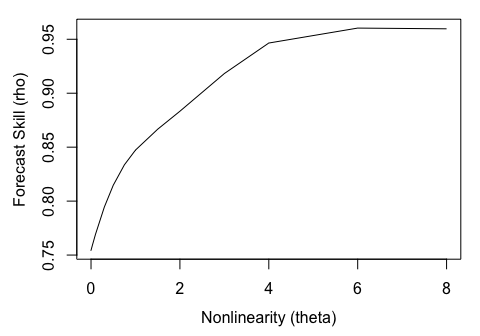Here, we can see that forecast skill substantially improves as theta increases, indicating the presence of nonlinear dynamics. Typically, we would expect forecast skill to decrease at high values of theta, because as theta, the local linear map can become overfitted to the nearest points. However, because the example data are observed without any error, even a very localized linear map can continue to produce good forecasts. By simulating the addition of a small amount of observational error, a more typical rho vs. theta plot can be achieved:

ts <- ts + rnorm(length(ts), sd = sd(ts) * 0.2)
smap_output <- s_map(ts, lib, pred, E = 2)
par(mar = c(4, 4, 1, 1), mgp = c(2.5, 1, 0))
plot(smap_output$theta, smap_output$rho, type = "l", xlab = "Nonlinearity (theta)",
ylab = "Forecast Skill (rho)")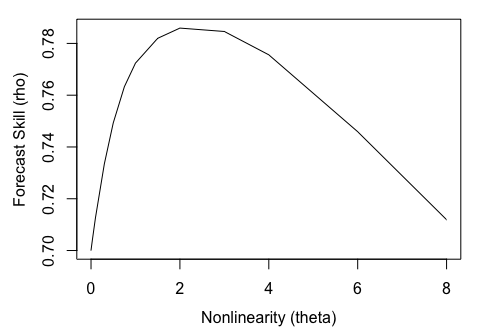## Generalized Takens’ Theorem

Instead of creating an attractor by taking lags of a single time series, it is possible to combine lags from different time series, if they are all observed from the same system (Sauer et al. 1991, Deyle and Sugihara 2011). The practical reality of applying EDM to systems with finite data, noisy observations, and stochastic influences means that such “multivariate” reconstructions can often be a better description of the true dynamics than “univariate” reconstructions

In rEDM, the block_lnlp function generalizes the simplex and s_map functions, allowing generic reconstructions to be used with either the simplex projection or S-map methods The main data input for block_lnlp is a matrix or data.frame of the time series observations, where each column is a separate time series with rows representing “simultaneous” observations. In addition to the standard parameters for simplex or s_map, block_lnlp contains parameters to specify which column is to be forecast (target_column) as well as which columns to use to construct the attractor (columns). For both parameters, either a numerical index or the column name can be given. Note that if lagged coordinates are intended to be used, they need to be manually created as separate columns in the matrix or data.frame.

### Example

We begin by loading an example dataset of time series and lags from a coupled 3-species model system. Here, the block_3sp variable is a 10-column data.frame with 1 column for time, and 3 columns for each of the variables (unlagged, $$t-1$$, and $$t-2$$ lags). Note that the lagged columns begin with NA values because there are no observations of the variables for times $$t < 1$$. In rEDM, vectors that include NA values are excluded if they would be used for computation, but predictions will still be made if only the observed values are missing (see section for more details).

data(block_3sp)
str(block_3sp)
## 'data.frame':    200 obs. of  10 variables:
##  $time : int 1 2 3 4 5 6 7 8 9 10 ... ##$ x_t  : num  -0.742 1.245 -1.918 -0.962 1.332 ...
##  $x_t-1: num NA -0.742 1.245 -1.918 -0.962 ... ##$ x_t-2: num  NA NA -0.742 1.245 -1.918 ...
##  $y_t : num -1.268 1.489 -0.113 -1.107 2.385 ... ##$ y_t-1: num  NA -1.268 1.489 -0.113 -1.107 ...
##  $y_t-2: num NA NA -1.268 1.489 -0.113 ... ##$ z_t  : num  -1.864 -0.482 1.535 -1.493 -1.119 ...
##  $z_t-1: num NA -1.864 -0.482 1.535 -1.493 ... ##$ z_t-2: num  NA NA -1.864 -0.482 1.535 ...

In order to correctly index into columns, block_lnlp has an option to indicate that the first column is actually a time index. When first_column_time is set to TRUE, a value of 1 for target_column now points to the first data column in the data.frame, as opposed to the time column (columns is similarly indexed).

In order to correctly index into columns, block_lnlp has an option to indicate that the first column is actually a time index. When first_column_time is set to TRUE}, a value of 1 for target_column will refer to the first data column in the data.frame, and skip the “time” column (the parameter columns is similarly indexed). If column names are used, then the first_column_time parameter is ignored, except to label the output.

lib <- c(1, NROW(block_3sp))
pred <- c(1, NROW(block_3sp))

cols <- c(1, 2, 4)  # c('x_t', 'x_t-1', 'y_t')
target <- 1  # 'x_t'

block_lnlp_output <- block_lnlp(block_3sp, lib = lib, pred = pred, columns = cols,
target_column = target, stats_only = FALSE, first_column_time = TRUE)

Note that the default value for the tp parameter is 1, indicating that predictions will be made for 1 time step into the future (i.e. the subsequent row of the input data). In some cases, the data may already be processed such that the predictions are already aligned in the same row, but a different column, in which case the tp parameter should be set to 0.

str(block_lnlp_output)
## List of 1
##  $:List of 4 ## ..$ params      :'data.frame': 1 obs. of  3 variables:
##   .. ..$embedding: int 1 ## .. ..$ tp       : num 1
##   .. ..$nn : num 4 ## ..$ embedding   : chr "1, 2, 4"
##   ..$model_output:'data.frame': 200 obs. of 4 variables: ## .. ..$ time    : num [1:200] 2 3 4 5 6 7 8 9 10 11 ...
##   .. ..$obs : num [1:200] 1.245 -1.918 -0.962 1.332 -0.817 ... ## .. ..$ pred    : num [1:200] NaN -1.226 -0.657 0.872 -1.754 ...
##   .. ..$pred_var: num [1:200] NaN 0.328 0.522 0.236 0.1 ... ## ..$ stats       :'data.frame': 1 obs. of  12 variables:
##   .. ..$num_pred : num 198 ## .. ..$ rho                : num 0.872
##   .. ..$mae : num 0.32 ## .. ..$ rmse               : num 0.434
##   .. ..$perc : num 0.889 ## .. ..$ p_val              : num 9.98e-79
##   .. ..$const_pred_num_pred: num 198 ## .. ..$ const_pred_rho     : num -0.539
##   .. ..$const_pred_mae : num 1.31 ## .. ..$ const_pred_rmse    : num 1.55
##   .. ..$const_pred_perc : num 0.394 ## .. ..$ const_p_val        : num 1

By setting stats_only to FALSE, the output is a list with the full model output. Because only 1 model (1 combination of input parameters) was run, the output is a list with 1 element. This element is actually another list with 4 named elements: params, a data.frame of the input parameters; embedding, a vector of the columns used to construct the model; model_output, a data.frame of the full set of observed and predicted values; and stats, a data.frame of the forecast statistics.

To compare the observed and predicted values, we must therefore index correctly into the output. We can then plot the observed and predicted values and see how well the model did relative to the expected 1:1 line.

observed <- block_lnlp_output[]$model_output$obs
predicted <- block_lnlp_output[]$model_output$pred

par(mar = c(4, 4, 1, 1), mgp = c(2.5, 1, 0), pty = "s")
plot_range <- range(c(observed, predicted), na.rm = TRUE)
plot(observed, predicted, xlim = plot_range, ylim = plot_range, xlab = "Observed",
ylab = "Predicted")
abline(a = 0, b = 1, lty = 2, col = "blue")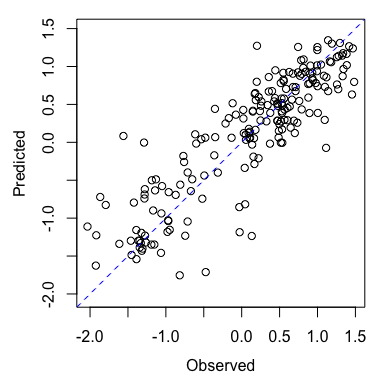## Causality Inference and Cross Mapping

One of the corollaries to the Generalized Takens’ Theorem is that it should be possible to cross predict or cross map between variables that are observed from the same system. Consider two variables, $$x$$ and $$y$$ that interact in a dynamic system. Then the univariate reconstructions based on $$x$$ or $$y$$ alone should uniquely identify the system state and and thus the corresponding value of the other variable.